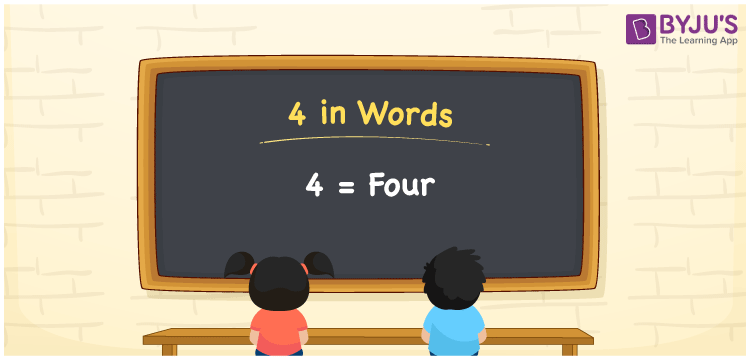# 4 in Words

4 in words is written as Four. In both the International System of Numerals and the Indian System of Numerals, 4 is written as Four. The number 4 is a Cardinal Number as it could represent some quantity. For example, “there are 4 notebooks in that bag”. 4 illustrates the number of notebooks.

 4 in Words Four Four in Number 4

## 4 in English Words

We write 4 in English Words using the letters of the English alphabet. Therefore, we spell 4 in English as “Four.”## How to Write 4 in Words?

To write 4 in words, we shall use the place value chart. In the place value chart, write 4 in the ones place and 0 in all the rest. Let us make a place value chart to write the number 4 in words.

 Hundreds Tens Ones 0 0 4

Thus, we can write the expanded form as

0 × Hundred + 0 × Ten + 4 × One

= 0 × 100 + 0 × 10 + 4 × 1

= 0 + 0 + 4

= 4

= Four

4 is the 4th natural number, the successor of 3 and the predecessor of 5.

4 in words – Four

• Is 4 an odd number? – No
• Is 4 an even number? – Yes
• Is 4 a perfect square number? – Yes; 22 = 4
• Is 4 a perfect cube number? – No
• Is 4 a prime number? – No
• Is 4 a composite number? – Yes

## Frequently Asked Questions on 4 in Words

Q1

### How to write 4 in English words?

4 in words is written as Four.
Q2

### How to spell 4?

The spelling of 4 is “Four.”
Q3

### Is 4 a Whole Number?

Yes, 4 is a Whole Number.